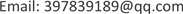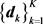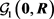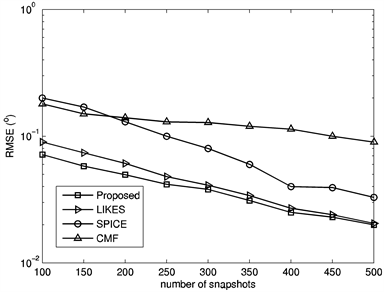﻿ 一种基于压缩感知的无需超参数的测向算法 A Hyperparameter-Free Direction-Finding Algorithm in the Compressive Sensing Framework

Hans Journal of Wireless Communications
Vol. 09  No. 01 ( 2019 ), Article ID: 28617 , 7 pages
10.12677/HJWC.2019.91001

A Hyperparameter-Free Direction-Finding Algorithm in the Compressive Sensing Framework

Xiangdong Ma, Ming Guo, Xiangyang Wu

Luoyang Electronic Equipment Test Center of China, Luoyang HenanReceived: Jan. 1st, 2019; accepted: Jan. 17th, 2019; published: Jan. 24th, 2019ABSTRACT

The fully automatic sparsity-parameter estimation algorithms do not require the user to make any hard decision (possibly via trial-and-error) on the values of the hyperparameters, making them more pragmatic in practice. This paper provides a unified interpretation of the existing approaches including covariance matrix fitting (CMF), sparse iterative covariance based estimation (SPICE) and likelihood-based estimation of sparse parameters (LIKES). The point of view taken is that they are all covariance-fitting-based algorithms under different statistical distances. Following this, we present a new covariance-fitting scheme trying to minimize one of the two asymmetrical Itakura-Saito distances. Simulations show that the proposed method appears to be preferable as it outperforms the aforementioned algorithms in general.

Keywords:Spectrum Management, Spectrum Monitoring, Direction Finding, Array Signal ProcessingCopyright © 2019 by author(s) and Hans Publishers Inc.1. 引言

2. 数学模型

$x\left(t\right)={\sum }_{m=1}^{M}{a}_{m}{s}_{m}\left(t\right)+n\left(t\right)$ (1)

${\left\{{\theta }_{k}\right\}}_{k=1}^{K}$ 为可以覆盖所有可能入射区域的栅格，并覆盖了(至少是近似覆盖了)上述M个信号的入射角度，相应的导向矢量为，其中， $K\gg N$ 。于是，式(1)可以重新表述为：

$x\left(t\right)={\sum }_{k=1}^{K}{d}_{k}{\stackrel{¯}{s}}_{k}\left(t\right)+n\left(t\right)$ (2)

$R=E\left\{x\left(t\right){x}^{\text{H}}\left(t\right)\right\}={\sum }_{k=1}^{K}{\sigma }_{k}{d}_{k}{d}_{k}^{\text{H}}+ϵI$ (3)

$R={\sum }_{k=1}^{K+N}{\sigma }_{k}{d}_{k}{d}_{k}^{\text{H}}$ (4)

$\stackrel{^}{R}=\frac{1}{L}{\sum }_{l=1}^{L}x\left[l\right]{x}^{\text{H}}\left[l\right]$ (5)

2.1. 协方差矩阵拟合算法(CMF)

$\begin{array}{l}\underset{{\left\{{\sigma }_{k}\right\}}_{k=1}^{K},ϵ}{\mathrm{min}}\left\{{d}_{\text{SE}}\left(\stackrel{^}{R},R\right)={‖\stackrel{^}{R}-\underset{k=1}{\overset{K}{\sum }}{\sigma }_{k}{d}_{k}{d}_{k}^{\text{H}}-ϵI‖}^{2}\right\}\\ \text{s}.\text{t}.\text{\hspace{0.17em}}\text{\hspace{0.17em}}{\left\{{\sigma }_{k}\right\}}_{k=1}^{K}\ge 0,\text{\hspace{0.17em}}\text{\hspace{0.17em}}{\sum }_{k=1}^{K}{\sigma }_{k}\le \lambda ,\text{\hspace{0.17em}}\text{\hspace{0.17em}}\lambda =-N{\gamma }_{N}+{\sum }_{n=1}^{N}{\gamma }_{n},\text{\hspace{0.17em}}\text{\hspace{0.17em}}ϵ\ge 0\end{array}$ (6)

$\begin{array}{l}\underset{{\left\{{\sigma }_{k}\right\}}_{k=1}^{K+N}}{\mathrm{min}}\left\{{d}_{\text{SE}}\left(\stackrel{^}{R},R\right)={‖\stackrel{^}{R}-\underset{k=1}{\overset{K+N}{\sum }}{\sigma }_{k}{d}_{k}{d}_{k}^{\text{H}}‖}^{2}\right\}\\ \text{s}.\text{t}.\text{\hspace{0.17em}}\text{\hspace{0.17em}}{\left\{{\sigma }_{k}\right\}}_{k=1}^{K+N}\ge 0\end{array}$ (7)

2.2. 基于稀疏迭代协方差的估计(SPICE)

SPICE算法  实际上是将两种高斯分布之间的Jeffreys距离(亦称为对称Kullback-Leibler距离)  最小化：

$\begin{array}{l}\underset{{\left\{{\sigma }_{k}\right\}}_{k=1}^{K+N}}{\mathrm{min}}\left\{{‖{R}^{-1/2}\left(\stackrel{^}{R}-R\right){\stackrel{^}{R}}^{-1/2}‖}^{2}=\text{tr}\left({R}^{-1}\stackrel{^}{R}\right)+\text{tr}\left({\stackrel{^}{R}}^{-1}R\right)-2N=2{d}_{KL}\left(\stackrel{^}{R},R\right)\right\}\\ \text{s}.\text{t}.\text{\hspace{0.17em}}\text{\hspace{0.17em}}\text{\hspace{0.17em}}R={\sum }_{k=1}^{K+N}{\sigma }_{k}{d}_{k}{d}_{k}^{\text{H}},\text{\hspace{0.17em}}\text{\hspace{0.17em}}\text{\hspace{0.17em}}{\left\{{\sigma }_{k}\right\}}_{k=1}^{K+N}\ge 0\end{array}$ (8)

2.3. 基于似然的稀疏参数估计(LIKES)

LIKES算法  使用的是高斯最大似然(GML)标准：

$\begin{array}{l}\underset{{\left\{{\sigma }_{k}\right\}}_{k=1}^{K+N}}{\mathrm{min}}\left\{{X}^{\text{H}}{R}^{-1}X+\text{ln}|R|\right\}\\ \text{s}.\text{t}\text{.}\text{\hspace{0.17em}}\text{\hspace{0.17em}}\text{\hspace{0.17em}}R={\sum }_{k=1}^{K+N}{\sigma }_{k}{d}_{k}{d}_{k}^{\text{H}},\text{\hspace{0.17em}}\text{\hspace{0.17em}}\text{\hspace{0.17em}}{\left\{{\sigma }_{k}\right\}}_{k=1}^{K+N}\ge 0\end{array}$ (9)

$\begin{array}{l}\underset{{\left\{{\sigma }_{k}\right\}}_{k=1}^{K+N}}{\mathrm{min}}\left\{{d}_{\text{IS}}\left(\stackrel{^}{R},R\right)=\text{tr}\left(\stackrel{^}{R}{R}^{-1}-I\right)-\text{ln}|\stackrel{^}{R}{R}^{-1}|\right\}\\ \text{s}.\text{t}\text{.}\text{\hspace{0.17em}}\text{\hspace{0.17em}}\text{\hspace{0.17em}}R={\sum }_{k=1}^{K+N}{\sigma }_{k}{d}_{k}{d}_{k}^{\text{H}},\text{\hspace{0.17em}}\text{\hspace{0.17em}}\text{\hspace{0.17em}}{\left\{{\sigma }_{k}\right\}}_{k=1}^{K+N}\ge 0\end{array}$ (10)

3. 本文提出的协方差矩阵拟合方案

$\begin{array}{l}\underset{{\left\{{\sigma }_{k}\right\}}_{k=1}^{K+N}}{\mathrm{min}}\left\{{d}_{\text{IS}}\left(R,\stackrel{^}{R}\right)=\text{tr}\left(R{\stackrel{^}{R}}^{-1}-I\right)-\text{ln}|R{\stackrel{^}{R}}^{-1}|\right\}\\ \text{s}.\text{t}\text{.}\text{\hspace{0.17em}}\text{\hspace{0.17em}}\text{\hspace{0.17em}}R={\sum }_{k=1}^{K+N}{\sigma }_{k}{d}_{k}{d}_{k}^{\text{H}},\text{\hspace{0.17em}}\text{\hspace{0.17em}}\text{\hspace{0.17em}}{\left\{{\sigma }_{k}\right\}}_{k=1}^{K+N}\ge 0\end{array}$ (11)

${d}_{\text{IS}}\left(R,\stackrel{^}{R}\right)=\text{tr}\left(R{\stackrel{^}{R}}^{-1}\right)-\text{ln}|R|+\text{const}={\sum }_{k=1}^{K+N}{\sigma }_{k}{d}_{k}^{\text{H}}{\stackrel{^}{R}}^{-1}{d}_{k}-\text{ln}|{\sum }_{k=1}^{K+N}{\sigma }_{k}{d}_{k}{d}_{k}^{\text{H}}|+\text{const}$ (12)

$\stackrel{¯}{R}=\stackrel{^}{\alpha }I+\stackrel{^}{\beta }\stackrel{^}{R}$ (13)

$\stackrel{^}{\rho }=\frac{1}{{L}^{2}}\underset{l=1}{\overset{L}{\sum }}{‖x\left[l\right]‖}^{4}-\frac{1}{L}{‖\stackrel{^}{R}‖}^{2}$

$\stackrel{^}{\nu }=\frac{\text{tr}\left(\stackrel{^}{R}\right)}{N}$

$\stackrel{^}{\beta }=1-\frac{\stackrel{^}{\alpha }}{\stackrel{^}{\nu }}$ (14)

$\begin{array}{l}\underset{{\left\{{\sigma }_{k}\right\}}_{k=1}^{K+N}}{\mathrm{min}}\left\{\underset{k=1}{\overset{K+N}{\sum }}{\sigma }_{k}{d}_{k}^{\text{H}}{\stackrel{^}{R}}^{-1}{d}_{k}-\text{ln}|\underset{k=1}{\overset{K+N}{\sum }}{\sigma }_{k}{d}_{k}{d}_{k}^{\text{H}}|\right\}\\ \text{s}.\text{t}.\text{\hspace{0.17em}}\text{\hspace{0.17em}}\text{\hspace{0.17em}}{\left\{{\sigma }_{k}\right\}}_{k=1}^{K+N}\ge 0\end{array}$ (15)

$c={\left[{d}_{1}^{\text{H}}{\stackrel{^}{R}}^{-1}{d}_{1},{d}_{2}^{\text{H}}{\stackrel{^}{R}}^{-1}{d}_{2},\cdots ,{d}_{K+N}^{\text{H}}{\stackrel{^}{R}}^{-1}{d}_{K+N}\right]}^{\text{T}}$ (16)

$p={\left[{\sigma }_{1},{\sigma }_{2},\cdots ,{\sigma }_{K+N}\right]}^{\text{T}}$ (17)

$D=\left[{d}_{1},{d}_{2},\cdots ,{d}_{K+N}\right]$ (18)

cvx_begin quiet

cvx_precision best

cvx_expert true

variable p(K+N,1)

minimize (c'*p-log_det(D*diag(p)*D'))

subject to

p >= zeros(K+N,1);

cvx_end

4. 计算机仿真

$\text{RMSE}=\sqrt{\frac{{\sum }_{n=1}^{300}\left[{\left({\stackrel{^}{\theta }}_{1,n}-{\theta }_{1}\right)}^{2}+{\left({\stackrel{^}{\theta }}_{2,n}-{\theta }_{2}\right)}^{2}+{\left({\stackrel{^}{\theta }}_{3,n}-{\theta }_{3}\right)}^{2}\right]}{900}}$ (19)Figure 1. RMSE versus varied SNRFigure 2. RMSE versus varied numbers of samples

5. 结论

A Hyperparameter-Free Direction-Finding Algorithm in the Compressive Sensing Framework[J]. 无线通信, 2019, 09(01): 1-7. https://doi.org/10.12677/HJWC.2019.91001

1. 1. ITU (2011) Spectrum Monitoring Handbook. ITU, Geneva.

2. 2. Capon, J. (1969) High-Resolution Frequency-Number Spectrum Analysis. Proceedings of the IEEE, 57, 1408-1418.
https://doi.org/10.1109/PROC.1969.7278

3. 3. Schmidt, R.O. (1986) Multiple Emitter Location and Signal Parameter Estimation. IEEE Transactions on Antennas and Propagation, 34, 276-280.
https://doi.org/10.1109/TAP.1986.1143830

4. 4. Malioutov, D., ?etin, M. and Willsky, A. (2005) A Sparse Signal Reconstruction Perspective for Source Localization with Sensor Arrays. IEEE Transactions on Signal Processing, 53, 3010-3022.
https://doi.org/10.1109/TSP.2005.850882

5. 5. Yardibi, T., Li, J., Stoica, P., et al. (2008) Sparsity Constrained Deconvolution Approaches for Acoustic Source Mapping. Journal of Acoustical Society of America, 123, 2631-2642.
https://doi.org/10.1121/1.2896754

6. 6. Stoica, P., Babu, P. and Li, J. (2011) SPICE: A Sparse Covariance-Based Estimation Method for Array Signal Processing. IEEE Transactions on Signal Processing, 59, 629-638.
https://doi.org/10.1109/TSP.2010.2090525

7. 7. Stoica, P. and Babu, P. (2012) SPICE and LIKES: Two Hyperparameter-Free Methods for Sparse-Parameter Estimation. Signal Processing, 92, 1580-1590.
https://doi.org/10.1016/j.sigpro.2011.11.010

8. 8. Tibshirani, R. (1996) Regression Shrinkage and Selection via the Lasso. Journal of Royal Statistical Society: Series B (Statistical Methodology), 58, 267-288.
https://doi.org/10.1111/j.2517-6161.1996.tb02080.x

9. 9. Gorodnitsky, I.F. and Rao, B.D. (1997) Sparse Signal Reconstruction from Limited Data Using FOCUSS: A Re-Weighted Minimum Norm Algorithm. IEEE Transactions on Signal Processing, 45, 600-616.
https://doi.org/10.1109/78.558475

10. 10. Cotter, S.F., Rao, B.D., Engan, K., et al. (2005) Sparse Solutions to Linear Inverse Problems with Multiple Measurement Vectors. IEEE Transactions on Signal Processing, 53, 2477-2488.
https://doi.org/10.1109/TSP.2005.849172

11. 11. Xu, D.Y., Hu, N., Ye, Z.F., et al. (2012) The Estimate for DOAs of Signals Using Sparse Recovery Method. Proceedings of the 37th IEEE International Conference on Acoustics, Speech, and Signal Processing, Kyoto, 2573-2576.
https://doi.org/10.1109/ICASSP.2012.6288442

12. 12. Ottersten, B., Stoica, P. and Roy, R. (1998) Covariance Matching Estima-tion Techniques for Array Signal Processing Applications. Digital Signal Processing, 8, 185-210.
https://doi.org/10.1006/dspr.1998.0316

13. 13. Kullback, S. (1997) Information Theory and Statistics. Dover Edition. Dover, New York.

14. 14. Bensaid, S. and Slock, D. (2012) Blind Audio Source Separation Exploiting Periodicity and Spectral Envelops. Pro-ceedings of International Workshop on Acoustic Signal Enhancement, Aachen.

15. 15. Vandenberghe, L., Boyd, S. and Wu, S.P. (1998) Determinant Maximization with Linear Matrix Inequality Constraints. SIAM Journal on Matrix Analysis and Applications, 19, 499-533.
https://doi.org/10.1137/S0895479896303430

16. 16. Landi, L., De Maio, A., De Nicola, S., et al. (2008) Knowledge-Aided Covariance Matrix Estimation: A MAXDET Approach. IET Radar, Sonar & Navigation, 3, 341-356.